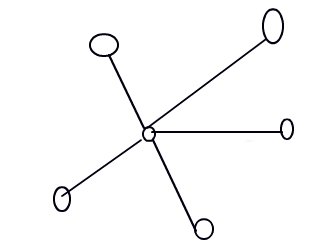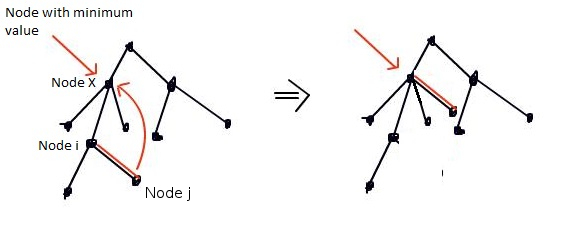# KINGSHIP - Editorial

Tester: Tasnim Imran Sunny
Editorialist: Lalit Kundu

SIMPLE-EASY

### PROBLEM:

Connect all the N(<=10^5) cities to make a connected graph in minimum cost. Cost of adding each edge is product of population of the two cities between whom edge is being added. All populations are given in the input.

### QUICK EXPLANATION:

Graph formed finally should be a tree. We connect all the other nodes to the node with smallest population which results in minimum cost.### EXPLANATION:

We need to add edges such that all the cities become connected. Connected is defined as “it should be possible from any city to reach any other city by a sequence of edges”.

There doesn’t exist a graph which is connected and has less than (N-1) edges (which is known as a tree).
So we will be constructing a tree finally with (N-1) edges.
Suppose we create some random tree with (N-1) edges. Now I pick two nodes i and j where Pi and Pj both are not the minimum and remove that edge from there and connect nodes x and j, where Px is minimum.

I have a change of ( Px * Pj ) - ( Pi * Pj ) which is less than zero since Px < Pi.So, we all nodes will be connected to the node with smallest value.
Pseudo Code:

``````n=input
value array=input
ans=0
for i=1 to N-1:
ans += value[i]*value
print ans
``````

### AUTHOR’S AND TESTER’S SOLUTIONS:

Author’s solution can be found here.
Tester’s solution can be found here.

7 Likes

@kuldeep992: You should have used long to avoid integer overflow.

1 Like

http://www.codechef.com/viewsolution/3429768

Why it got wrong?

Isn’t it a minimum spanning tree problem? If so, can we solve it using kruskal or prim algo.

can anyone explain me where is integer overflowing in my code ?
http://www.codechef.com/viewplaintext/3426847

Can kruskal’s and prim algorithm be used ?

to avoid integer overflow use ’ long long ’ . I had got a WA while using ‘int’ . and the problem is of
'ad-hoc ’ category which means you do not require to apply any special algorithm to get it solved .

please explain the case n=1…why isn’t the ans=0 for that?

what is the answer if input:
1
2
5 5

why can’t we use long n, long vector and long long sum??

can you tell me why i got wrong answer…
http://www.codechef.com/viewsolution/3428578

http://www.codechef.com/viewsolution/3427213

sme here!! can u tell d reson 4r it’s faliure?

long is 32 bit. You are getting integer overflow in your code.

2 Likes

I think you can user Prim’s algorithm, because of it’s implementation if you start from vertex with min Pi, but not Kruskal’s…

it is `sum+=a[i]*min;`

try

``````1
3
1000000 1000000 1000000
``````

i got it. thanks !Y cant kruskal be used ?

Complexity of Kruskal’s is O(ElogV), while of this algorithm is linear. I guess it might result in TLE. Morever this algorithm is very easy to implement unlike Kruskal.

No need to use tree or graph…
A simple solution

#include<bits/stdc++.h>
using namespace std;
int main()
{
long long int T,N;
cin>>T;
while(T–)
{
cin>>N;
long long int arr[N];
for(long long int i=0;i<N;i++)
{
cin>>arr[i];
}
sort(arr,arr+N);
long long int sum=0;
for(long long int i=1;i<N;i++)
{
sum+=arr[i]*arr;
}
cout<<sum<<endl;
}
}

Why no one has mentioned the graph would be STAR? Such an easy problem!!!